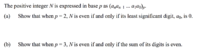# Proofs Relating to Integer Representation in Various Bases

#### furthermathishard

##### New member
Hi ya'll, I'm currently really confused about a question that I met in a math exercise that's given by my teacher related to integers in other bases.
Posted below is the original question:I have already solved (probably incorrectly?) part (a), and am now attempting to write the proof for part (b)
I understand that I need to dissociate the base 3 into the factors that make it up, for instance
If N is even, then (anan-1 ... a1a0)3 = (2m)10
= 3^m x an + 3^m-1 x an-1...3a1+a0
= 3(3^m-1 x an + 3^m-2 x an-1...a1) + a0
Then I separated it into two cases:
1) if the sum of the terms in the bracket is odd
3 x even = even
even + odd/even = even
2) if the sum of the terms in the bracket is even
3 x odd = odd
odd + odd = even
odd + even = odd
so a0 is odd?

I have no idea how to continue from this point on, and this is only the proof from one direction ://

#### Jomo

##### Elite Member
Before we move onto part b can we please see your work for part a?

#### JeffM

##### Elite Member
I reiterate jomo's point, but ask as well did you try attacking part b by weak induction?

#### MarkFL

##### Super Moderator
Staff member
For part (b) I would begin by stating:

$$\displaystyle N=\sum_{k=0}^n\left(a_k(2+1)^k\right)$$

And then using the binomial theorem:

$$\displaystyle N=\sum_{k=0}^n\left(a_k\sum_{j=0}^k\left({k \choose j}2^{j}\right)\right)$$

$$\displaystyle N=\sum_{k=0}^n\left(a_k\left(1+\sum_{j=1}^{k-1}\left({k \choose j}2^{j}\right)\right)\right)$$

Can you proceed?

•furthermathishard

#### MarkFL

##### Super Moderator
Staff member
To follow up, we may write:

$$\displaystyle N=2\sum_{k=0}^{n}\left(a_k\sum_{j=1}^{k-1}\left({k \choose j}2^{j-1}\right)\right)+\sum_{k=0}^{n}\left(a_k\right)$$

The parity of the sum of an even number (the first sum as it is a multiple of 2) and the sum of the digits (the second sum) will only be even if the second sum is even.

•furthermathishard

Nice paper!